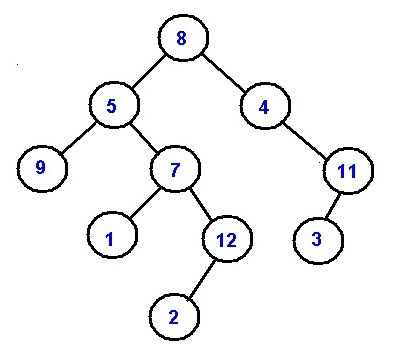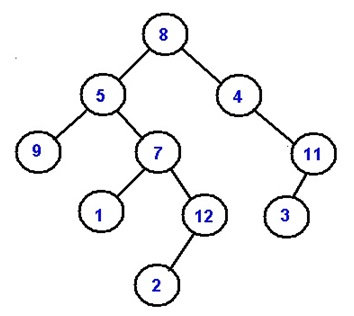Q:

# All Root to Leaf Paths

Given a Binary Tree of size N, write a program that prints all the possible paths from root node to the all the leaf node's of the binary tree.

Example:

Let us the tree be like following:All possible root to leaf paths in this tree is:

```    8->5->9
8->5->7->1
8->5->7->12->2
8->4->11->3```

To print all the root to leaf paths we have used recursive approach.

The idea is to maintain a list of nodes on the paths and to print the list while leaf node is reached.

Algorithm:

Pre-requisite:

Input binary tree root, list a

```    FUNCTION printpathrecursively(Node* current_node, list  a){
1.  base case
IF(current_node ==NULL)
return;
END IF
2.  append current_node ->data to the list;
IFcurrent_nodeis leaf node //leaf node reached
Print the list; //printing the root to leaf path
END IF
3.  printpathrecursively(current_node->left,a);//recur for left subtree
printpathrecursively(current_node->right,a);//recur for right subtree
END FUNCTION
```

In the main function create an empty list a, And call printpathrecursively(root, a);

Example with explanation:Nodes are represented by their respective values.

```    In main we call printpathrecursively(root, a)
----------------------------------------------

printpathrecursively(8, a)
current node not NULL
append 8 to list a
list: 8
current node is not leaf node
call to printpathrecursively(8->left, a)
call to printpathrecursively(8->right, a)
----------------------------------------------

printpathrecursively(8->left, a):
=printpathrecursively(5, a)
current node not NULL
append 5 to list a
list: 8,5
current node is not leaf node
call to printpathrecursively(5->left, a)
call to printpathrecursively(5->right, a)
----------------------------------------------

printpathrecursively(5->left, a):
=printpathrecursively(9, a)
current node not NULL
append 9 to list a
list: 8,5, 9
current node is leaf node
Print the list
call to printpathrecursively(9->left, a)
call to printpathrecursively(9->right, a)
call to printpathrecursively(9->left, a)
----------------------------------------------

=printpathrecursively(NULL, a)
current nodeNULL

At printpathrecursively(9, a)
call to printpathrecursively(9->right, a)
----------------------------------------------

=printpathrecursively(NULL, a)
current node NULL
At printpathrecursively(9, a)
Control reaches end of the void function
Thus control returns to the calleefunction  printpathrecursively(5, a)
printpathrecursively(5->right, a):
----------------------------------------------

=printpathrecursively(7, a)
current node not NULL
append 7 to list a
list: 8, 5, 7 //Don’t think list will be 8, 5, 9, 7.
List at this function was 8, 5 not 8, 5, 9
//List has not been passed by referenced
current node is not leaf node

call to printpathrecursively(7->left, a)
call to printpathrecursively(7->right, a)
This can be carried out until control gets back to main
which called function printpathrecursively(root, a)
```

You can do rest by your own to have much more clear idea about how the program is actually working.

C++ Implementation:

``````#include <bits/stdc++.h>
using namespace std;

//tree node
class Node{
public:
int data;
Node *left;
Node *right;
};

void print(vector<int> a){
for(int i=0;i<a.size();i++){
if(i==a.size()-1)
cout<<a[i];
else
cout<<a[i]<<"->";
}
}

void printpathrecursively(Node* root,vector<int> a){
if(root==NULL) //base case
return ;
a.push_back(root->data);
if(!root->left && !root->right){ //leaf node reached
print(a);
cout<<endl;
}
printpathrecursively(root->left,a);//recur for left subtree
printpathrecursively(root->right,a);//recur for right subtree
}

void printPaths(Node* root)
{
vector<int> a;
printpathrecursively(root,a);
}

//creating new nodes
Node* newnode(int data){
Node* node = (Node*)malloc(sizeof(Node));
node->data = data;
node->left = NULL;
node->right = NULL;
return(node);
}

int main() {
//**same tree is builted as shown in example**
cout<<"tree in the example is build here"<<endl;
//building the tree like as in the example
Node *root=newnode(8);
root->left= newnode(5);
root->right= newnode(4);
root->right->right=newnode(11);
root->right->right->left=newnode(3);
root->left->left=newnode(9);
root->left->right=newnode(7);
root->left->right->left=newnode(1);
root->left->right->right=newnode(12);
root->left->right->right->left=newnode(2);

cout<<"Printing all root to leaf paths......"<<endl;
printPaths(root);

return 0;
}
``````

Output

```tree in the example is build here
Printing all root to leaf paths......
8->5->9
8->5->7->1
8->5->7->12->2
8->4->11->3 ```